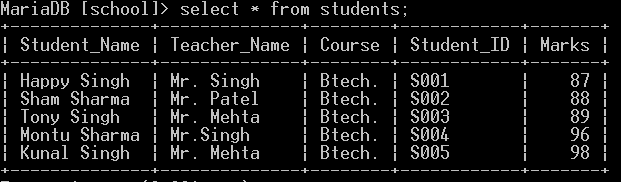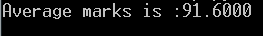GFG App
Open AppBrowser
Continue

# How to Compute the Average of a Column of a MySQL Table Using Python?

A MySQL connector is needed to generate a connection between Python and the MySQL server. Here we will import mysql.connector library to get the average of the specified column in the given database.

If you need to know how to install MySQL, see How to Install MySQL in Python 3.

### Average Function of SQL

SQL AVG() function returns the average of values of a numeric column in a table. It is generally used with the WHERE clause.

AVG() Function Syntax

SELECT AVG(column_name)
FROM table_name
WHERE condition;

Database used:Students Table in school database

#### Steps to be followed:

• So we first must import mysql.connector . Once that is imported, we gain connection to the MySQL database using the mysql.connector.connect() function.
• We then have to create a cursor for the table.
• Next, we execute our function to find the average of the Marks column of the Students table using the cursor.execute() function. Inside of this function, we place in the line, “SELECT AVG(Marks) AS average FROM students”.
• We then create a variable named rows and set it equal to cursor.fetchall().
• We then use a for loop and print out i, which represents the average of the Marks column.
• We then close the database once we’ve done what we’ve needed to.
• And by this is we can find the average of all the rows of a column in a MySQL table using Python.

Implementation:

Program to find Average using MySQL connector in Python 3.

## Python3

 `import` `mysql.connector`   `# database connection` `connection ``=` `mysql.connector.connect(` `    ``host``=``"localhost"``, user``=``"root"``, ` `      ``password``=``"``", database="``school")` `cursor ``=` `connection.cursor()`   `# queries for retrievint all rows` `retrieve ``=` `"Select AVG(Marks) AS average from students;"`   `# executing the queries` `cursor.execute(retrieve)` `rows ``=` `cursor.fetchall()` `for` `i ``in` `rows:` `    ``print``(``"Average marks is :"` `+` `str``(i[``0``]))`     `# committing the connection then closing it.` `connection.commit()` `connection.close()`

Output:Output of Marks Column Average of Students Table.

My Personal Notes arrow_drop_up